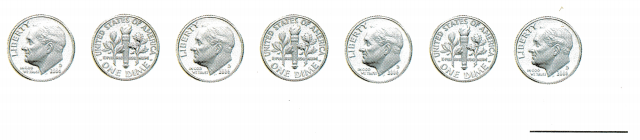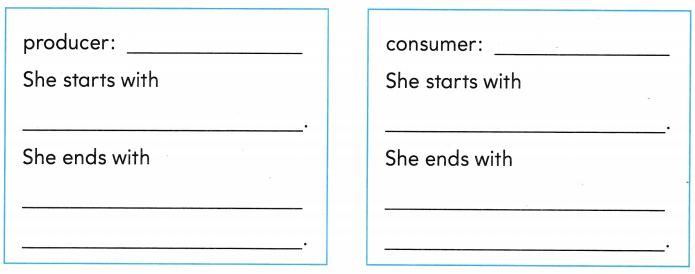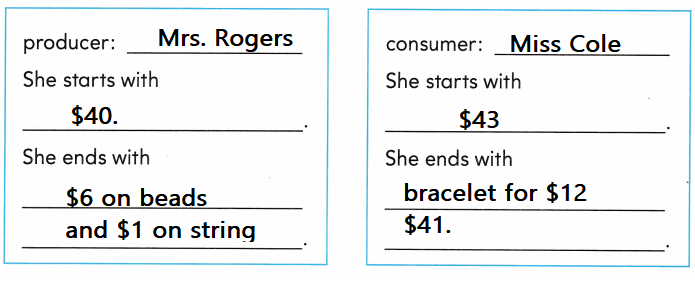Refer to our Texas Go Math Grade 2 Answer Key Pdf to score good marks in the exams. Test yourself by practicing the problems from Texas Go Math Grade 2 Unit 6 Assessment Answer Key.

Concepts and Skills

Use the coins to answer the question. TEKS 2.11.B

Question 1.
Grady gets one dime each week for 7 weeks. He saves 5 dimes and spends 2 dimes. How much money does he have now?Name the producer and consumer.
List what they started with and what they end with. TEKS 2.11.F
Explanation:
The producer and consumer are same in the above problem
They started with 7 and end With 5

Question 2.
Mrs. Rogers has $40. She spends$6 on beads and $1 on string. She makes a bracelet. Miss Cole has$43 and buys the bracelet for $12.Explanation:The above information is completed in the given table. Question 3. How much did Mrs. Rogers spend on things needed to make a bracelet? TEKS 2.11.F Answer:$7
Explanation:
She spends $6 on beads and$1 on string
$7is the amount that Mrs. Rogers spend on things needed to make a bracelet. Texas Test prep Fill in the bubble for the correct answer choice. Question 4. Aaron has$22 in the bank. He makes a deposit of $5. How much money does he have in the bank now? TEKS 2.11.C$17$27$23
Answer:  $27 Explanation:$27 money does he have in the bank now
22 + 5 = 27

Question 5.
Cathy has $30 in the bank. She makes a withdrawal of$4. How much money does she have in the bank now? TEKS 2.11.C$43$26$34 Answer:$26
Explanation:
$26 money does she have in the bank now 30 – 4 = 26$

Question 6.
Riley gets 2 dimes and I nickel each week for 3 weeks. He spends 20$and saves the rest. How much money does he have now? TEKS 2.11.B55¢40¢65¢ Answer: 55¢ Explanation: 1 dime = 10 cents 2 x 10 = 20 1 nickel = 5 cents 20 + 5 = 25 x 3 = 75¢ He spends 20$ and saves the rest
75 – 25 = 55¢

Question 7.
Shandra has 67¢. She wants to buy a puzzle that costs 95¢. How much money does she need to borrow? TEKS 2.11.D32¢28¢162¢
Explanation:
Shandra has 67¢. She wants to buy a puzzle that costs 95¢.
95 – 67 = 28 ¢.

Texas Test Prep

Question 8.
Tasha lends $6 to Joe. Joe plans to pay$2 to Tasha each week. How long will it take for Joe to pay back the $6? TEKS 2.11.E2 weeks8 weeks3 weeks Answer: 3 weeks Explanation: 3 weeks it take for Joe to pay back the$6

Question 9.
Cody wants to buy a gift for his brother that costs 8 dollars. If he saves 4 dollars each week, how long will it take him to save enough money to buy the gift? TEKS 2.11.A2 weeks6 weeks4 weeks
Explanation:
2 weeks it take him to save enough money to buy the gift
2 x 4 = 8

Question 10.
Mr. Baxter has $50 in the bank. He makes 4 deposits of$5. How much money is in the bank now? TEKS 2.11.C$59$70$41 Answer:$70
Explanation:
50 + 4 x 5 = 20
50 + 20 = 70$money is in the bank now Question 11. Rex lends one dollar to George. George plans to pay Rex 25c each week. How long will it take for George to pay back the dollar? TEKS 2.11.E1 weeks4 weeks2 weeks Answer: 4 weeks 25 x 4 = 100 Explanation: One cent is the same as one penny. One hundred cents make a dollar or 1 cent = 1/100 dollar. Question 12. Judy asked if borrowing money is like saving money. What would you tell her? TEKS 2.11.B, 2.11.D Answer: No, Explanation: Borrowing does not mean saving the money we have return it back if we borrow. Use the information to complete. Question 13. Write and draw to show what Becky does with her money each week. Remember: She should have$20 at the end. TEKS 2.11.A, 2.11.B

• At the beginning of the first week, Becky has $12. • She earns$4 each week.
• She spends some money each week.
• At the end of 4 weeks, she has $20. How much money did Becky save during the 4 weeks? ____ Explain why you think your way of solving this problem worked. Answer:$20
Explanation:
$20 money has Becky save during the 4 weeks • At the beginning of the first week, Becky has$12.
• She spends some money each week.
• She earns $4 each week. • At the end of 4 weeks, she has$20.
4 x 4 = 16
16 + 12 = 28
he spends $2 per week so,$20 is remaining.
Scroll to Top